Learn all Concepts of Polynomials Class 9 (with VIDEOS). Check - Polynomials Class 9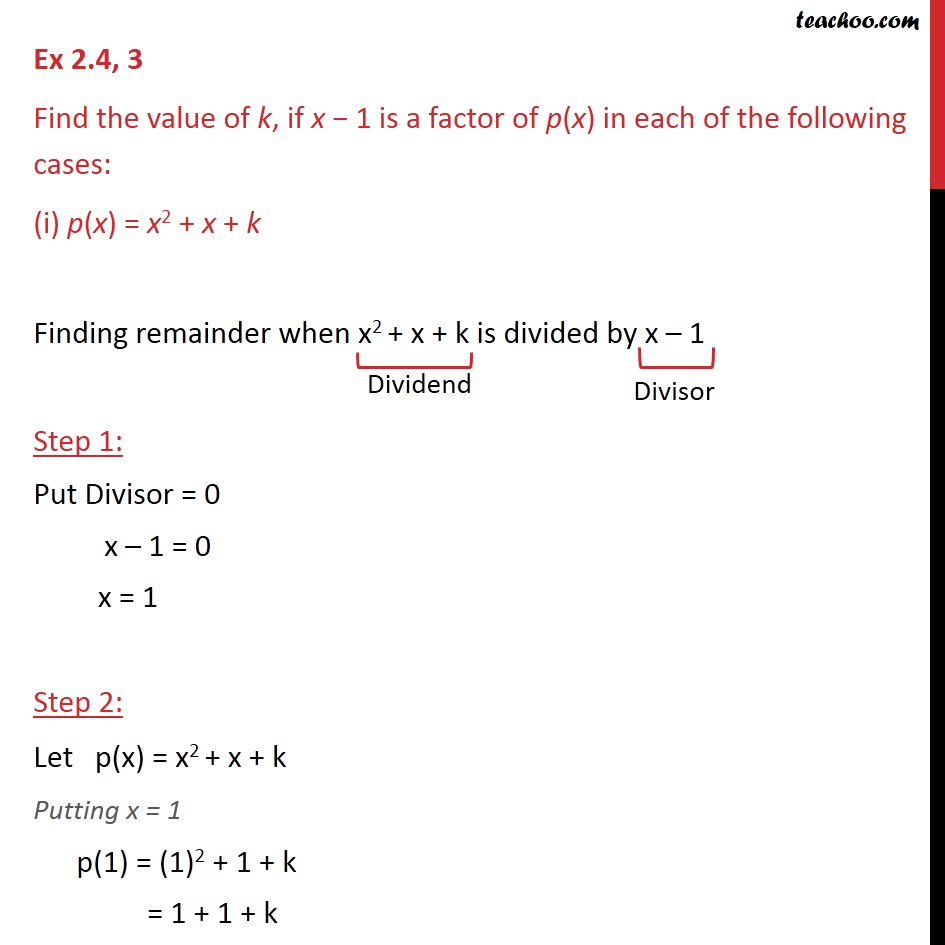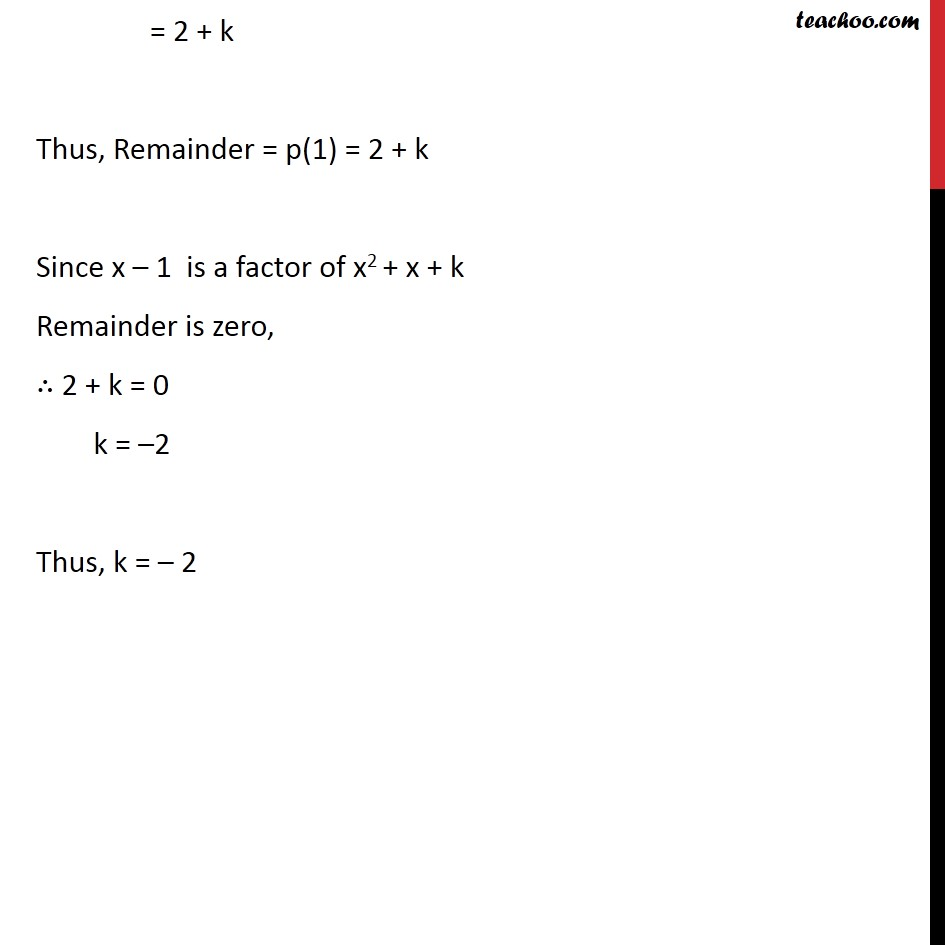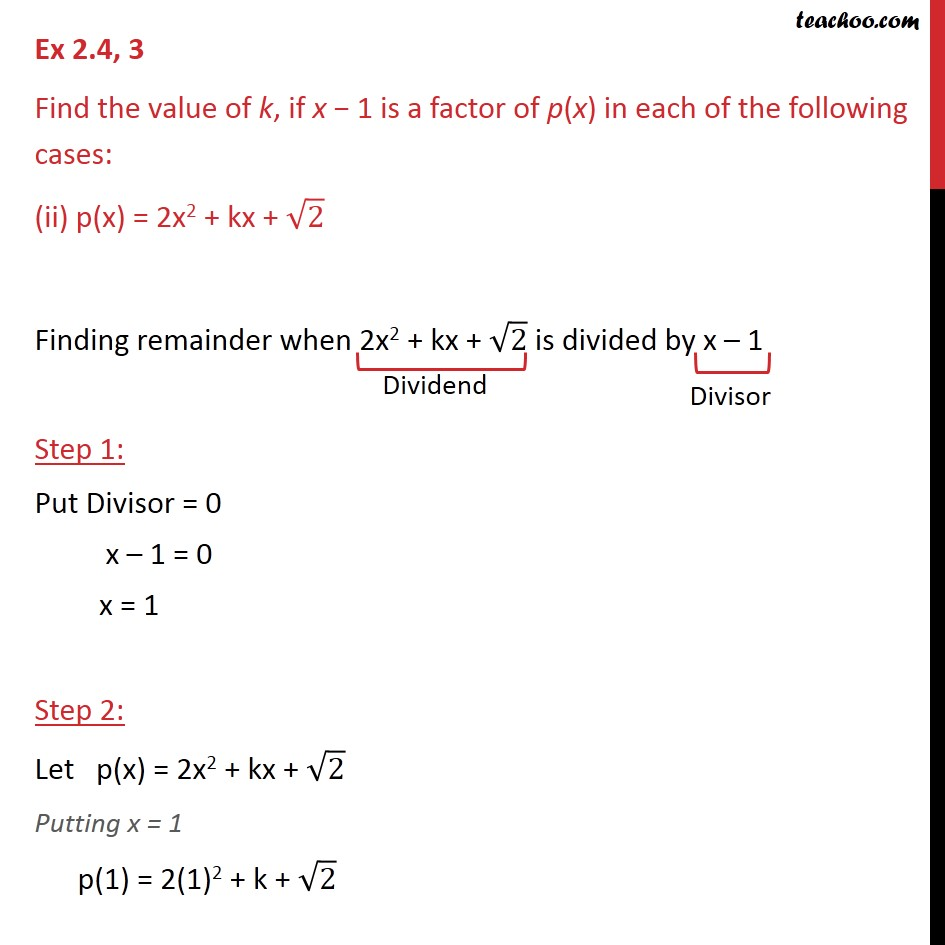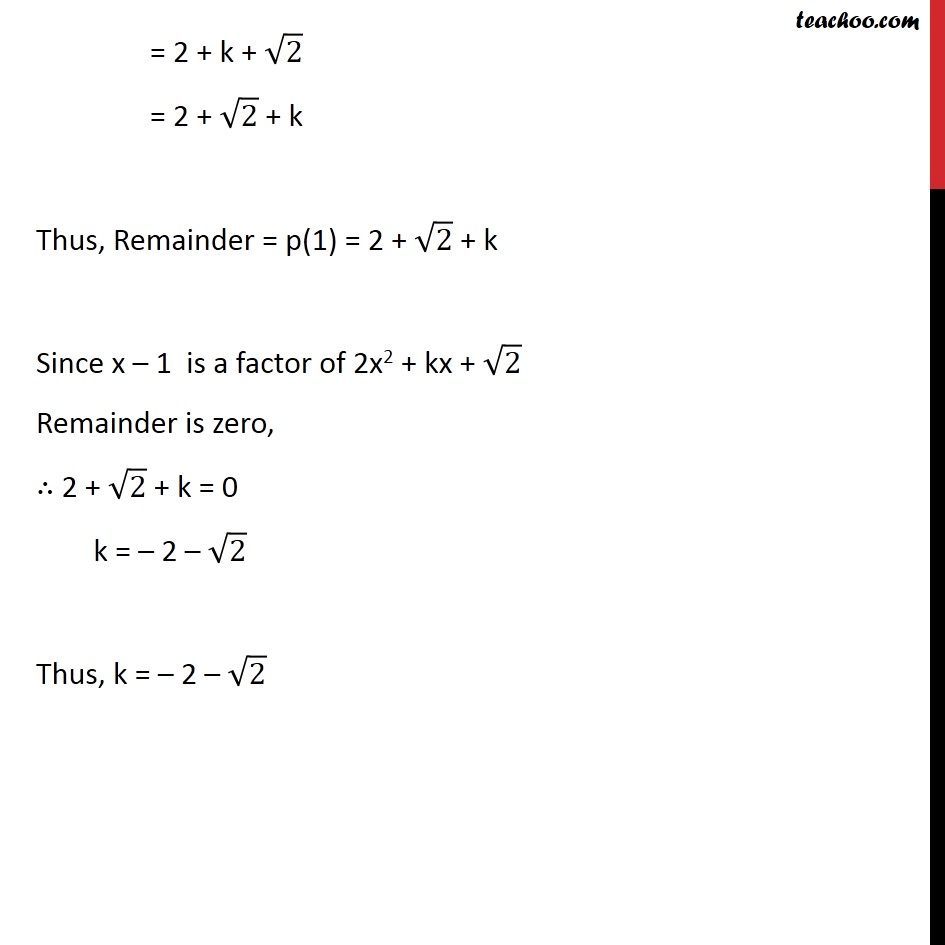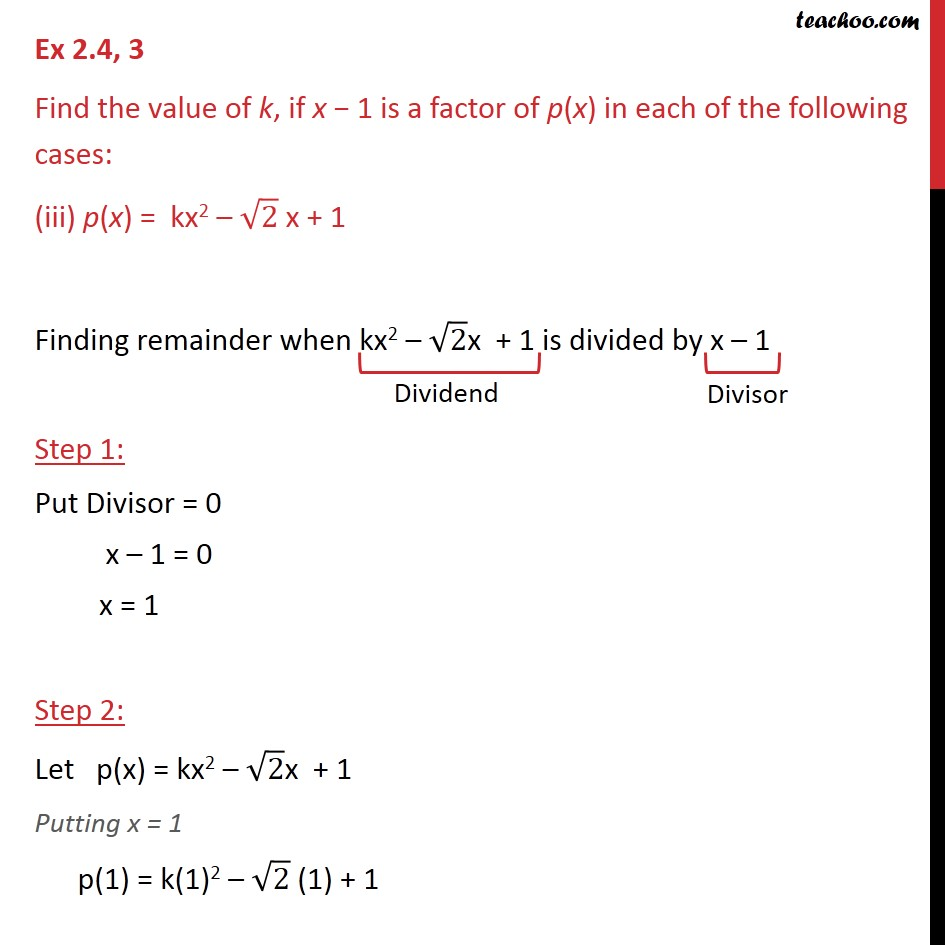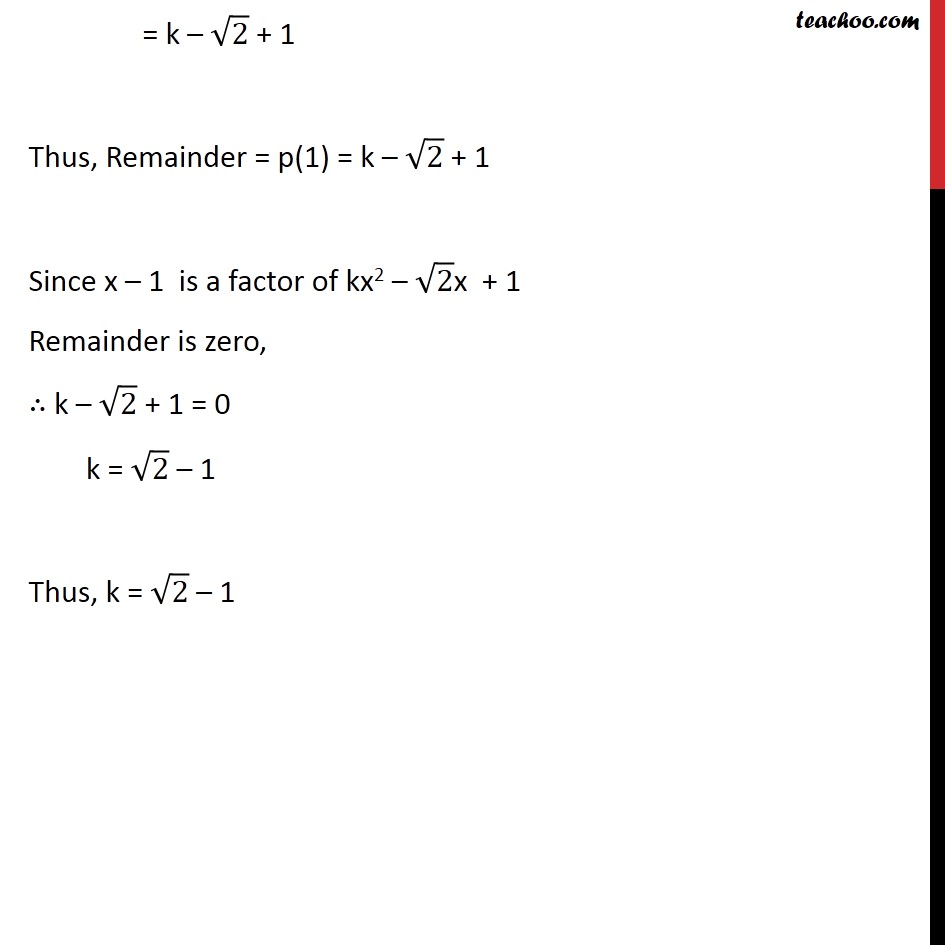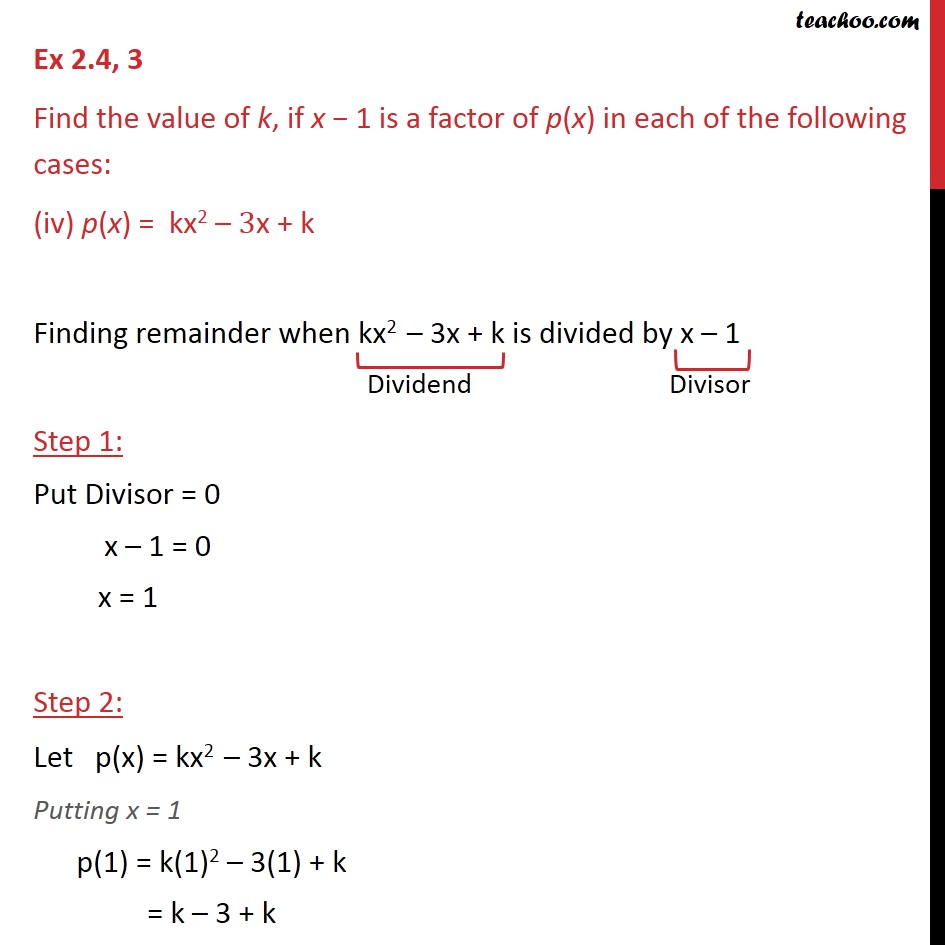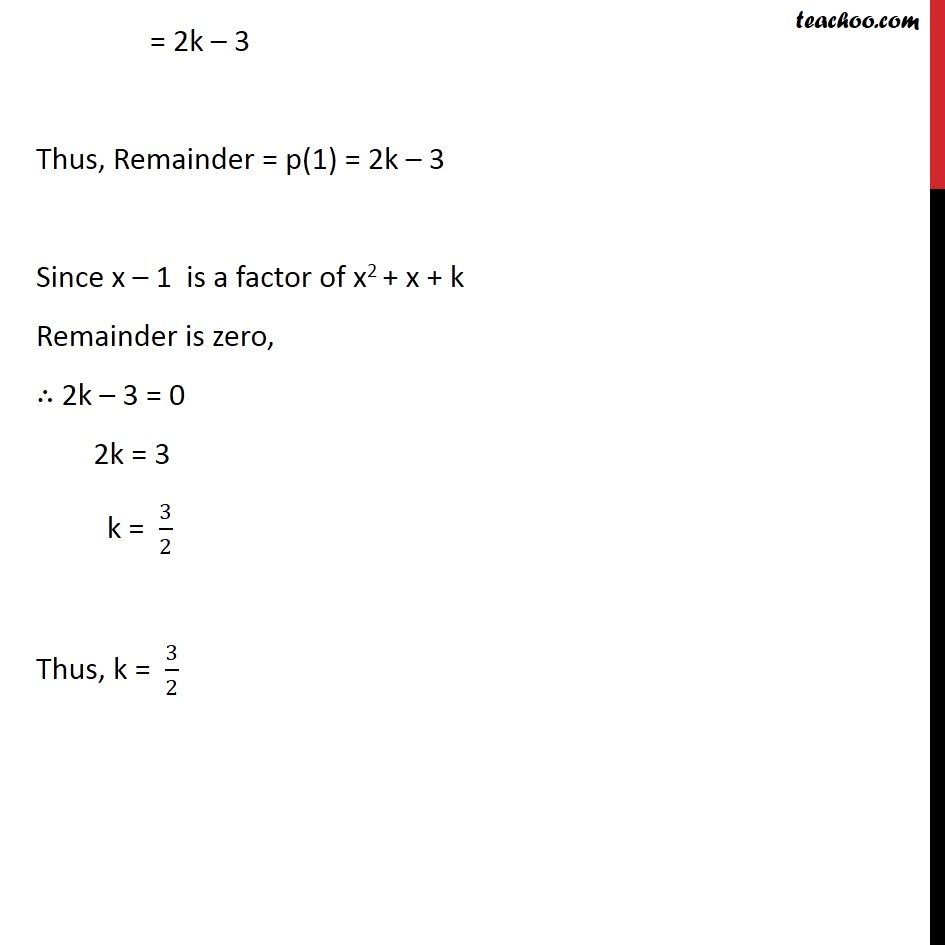1. Chapter 2 Class 9 Polynomials
2. Serial order wise
3. Ex 2.4

Transcript

Ex 2.4, 3 Find the value of k, if x 1 is a factor of p(x) in each of the following cases: (i) p(x) = x2 + x + k Finding remainder when x2 + x + k is divided by x 1 Step 1: Put Divisor = 0 x 1 = 0 x = 1 Step 2: Let p(x) = x2 + x + k Putting x = 1 p(1) = (1)2 + 1 + k = 1 + 1 + k = 2 + k Thus, Remainder = p(1) = 2 + k Since x 1 is a factor of x2 + x + k Remainder is zero, 2 + k = 0 k = 2 Thus, k = 2 Ex 2.4, 3 Find the value of k, if x 1 is a factor of p(x) in each of the following cases: (ii) p(x) = 2x2 + kx + 2 Finding remainder when 2x2 + kx + 2 is divided by x 1 Step 1: Put Divisor = 0 x 1 = 0 x = 1 Step 2: Let p(x) = 2x2 + kx + 2 Putting x = 1 p(1) = 2(1)2 + k + 2 = 2 + k + 2 = 2 + 2 + k Thus, Remainder = p(1) = 2 + 2 + k Since x 1 is a factor of 2x2 + kx + 2 Remainder is zero, 2 + 2 + k = 0 k = 2 2 Thus, k = 2 2 Ex 2.4, 3 Find the value of k, if x 1 is a factor of p(x) in each of the following cases: (iii) p(x) = kx2 2 x + 1 Finding remainder when kx2 2x + 1 is divided by x 1 Step 1: Put Divisor = 0 x 1 = 0 x = 1 Step 2: Let p(x) = kx2 2x + 1 Putting x = 1 p(1) = k(1)2 2 (1) + 1 = k 2 + 1 Thus, Remainder = p(1) = k 2 + 1 Since x 1 is a factor of kx2 2x + 1 Remainder is zero, k 2 + 1 = 0 k = 2 1 Thus, k = 2 1 Ex 2.4, 3 Find the value of k, if x 1 is a factor of p(x) in each of the following cases: (iv) p(x) = kx2 3x + k Finding remainder when kx2 3x + k is divided by x 1 Step 1: Put Divisor = 0 x 1 = 0 x = 1 Step 2: Let p(x) = kx2 3x + k Putting x = 1 p(1) = k(1)2 3(1) + k = k 3 + k = 2k 3 Thus, Remainder = p(1) = 2k 3 Since x 1 is a factor of x2 + x + k Remainder is zero, 2k 3 = 0 2k = 3 k = 3/2 Thus, k = 3/2

Ex 2.4

Chapter 2 Class 9 Polynomials
Serial order wise